•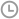发布时间：2019-07-04 17:06:13
• |
• 出处：PDF猫
• |
• 阅读量：147

Sumproduct函数的本职工作是返回两组数乘积的和，如：

=SUMPRODUCT(B2:B7,C2:C7)>>>相关阅读：你知道SUMPRODUCT函数的套路吗?<<<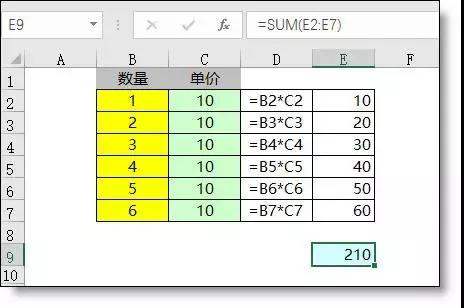=SUMPRODUCT((A2:A5=B8)*1)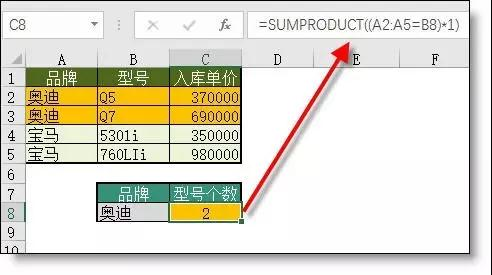=SUMPRODUCT((B3:B9="A")*C3:C9)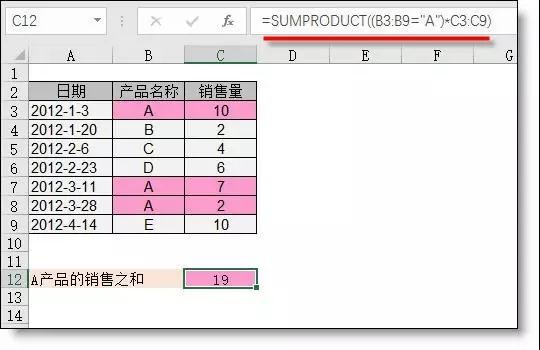=SUMPRODUCT((B3:B9="A")*(MONTH(A3:A9)=3))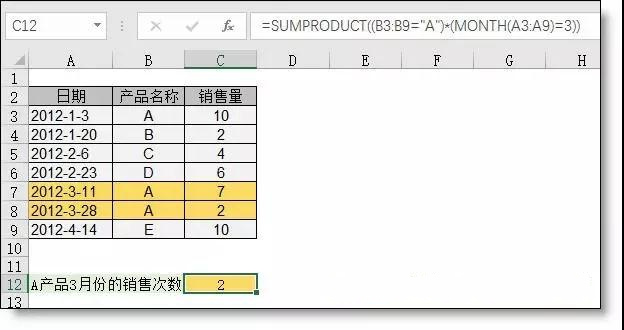=SUMPRODUCT((B3:B9="A")*(MONTH(A3:A9)=3)*C3:C9)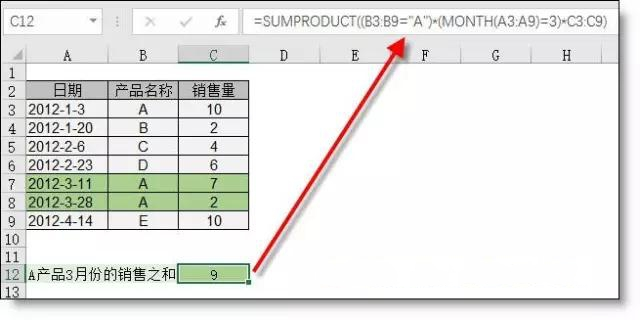=SUMPRODUCT((A2:A5=A8)*(B2:B5=B8)*C2:C5)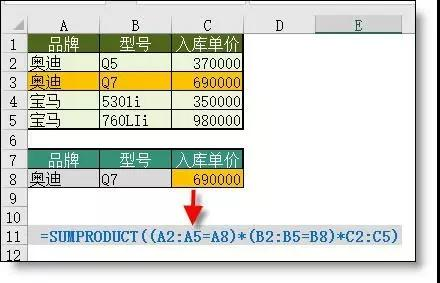=SUMPRODUCT(1/COUNTIF(A2:A7,A2:A7))=SUMPRODUCT(B2:B10*1)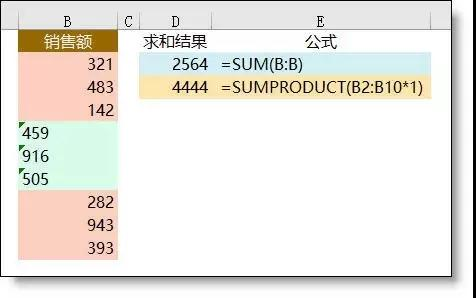PDF猫转换器

PDF猫转换器